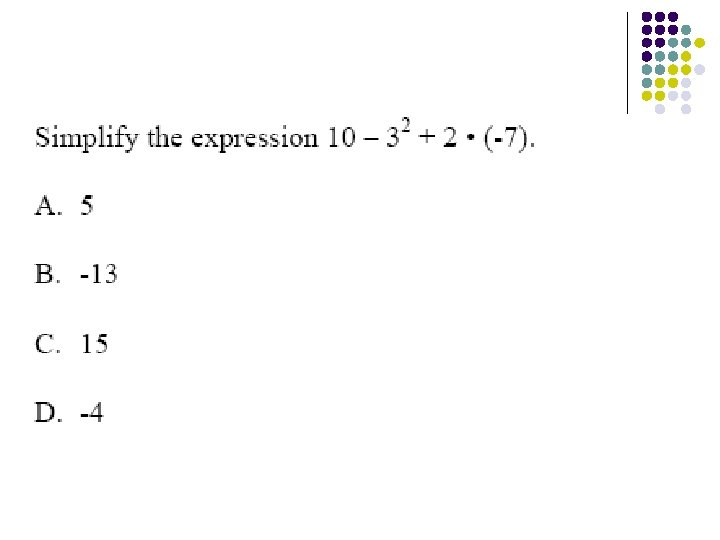# Exponents Standard Exponent Tells how many times a

• Slides: 63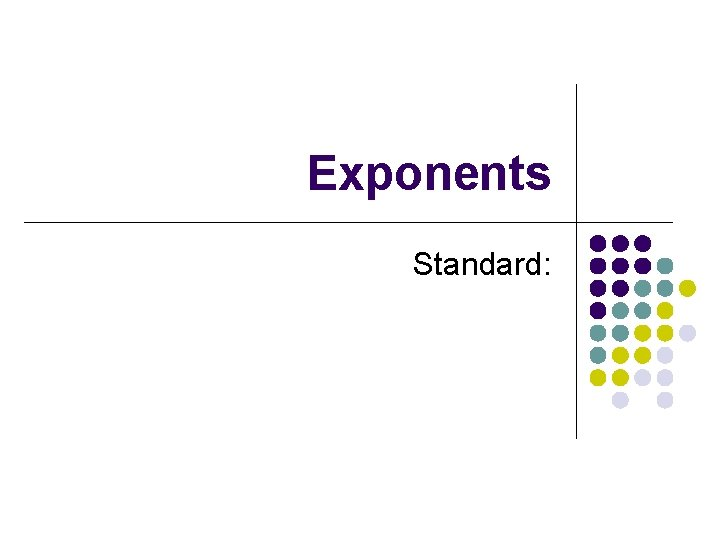Exponents Standard: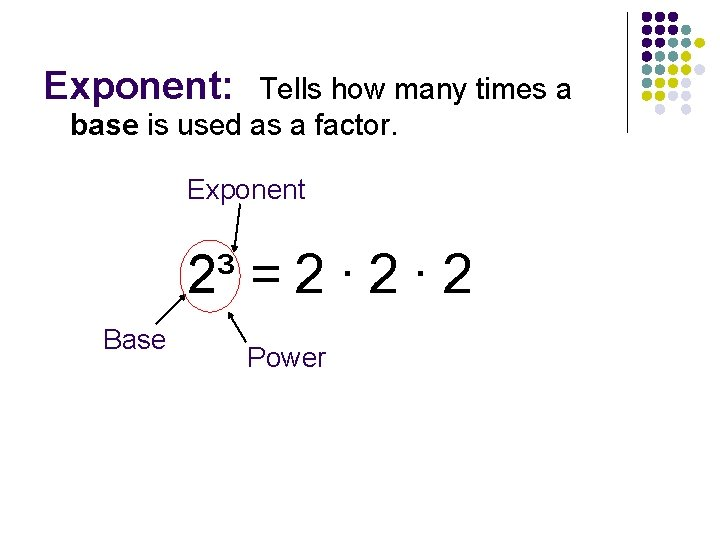Exponent: Tells how many times a base is used as a factor. Exponent 2³ = 2 ∙ 2 Base Power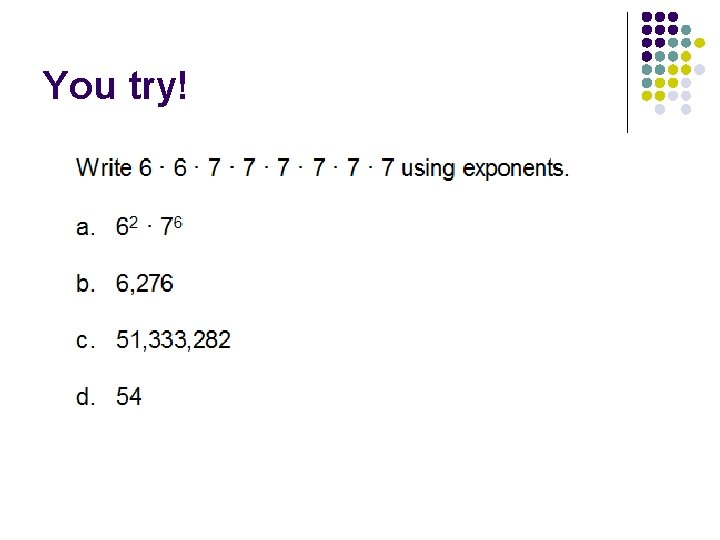You try!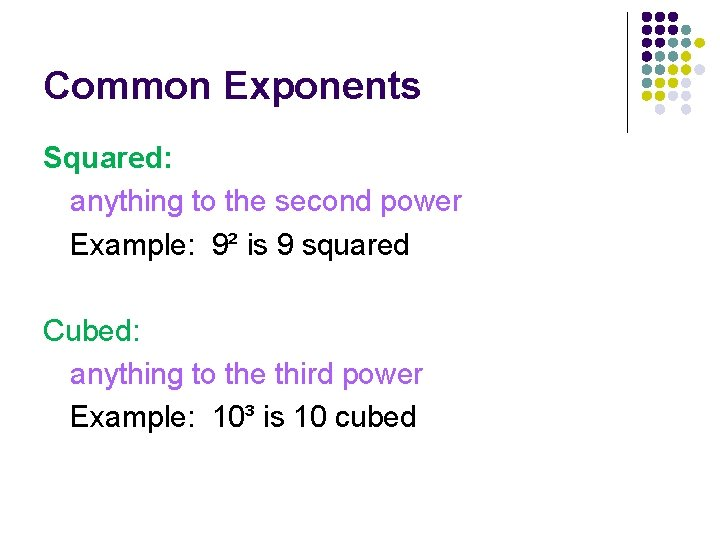Common Exponents Squared: anything to the second power Example: 9² is 9 squared Cubed: anything to the third power Example: 10³ is 10 cubedZero Power: anything to the zero power is ONE! Example: 8⁰ = 1 (-24)⁰ = 1 2, 500, 050⁰ = 1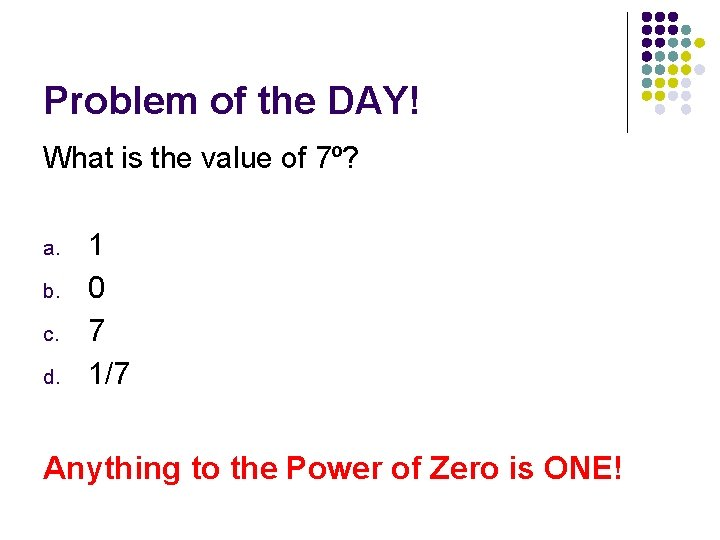Problem of the DAY! What is the value of 7⁰? a. b. c. d. 1 0 7 1/7 Anything to the Power of Zero is ONE!Remember! The exponent tells you how many times you will multiply the same number by itself! Even when it is negative! For example: (-5)³ = (-5) ∙ (-5) = 125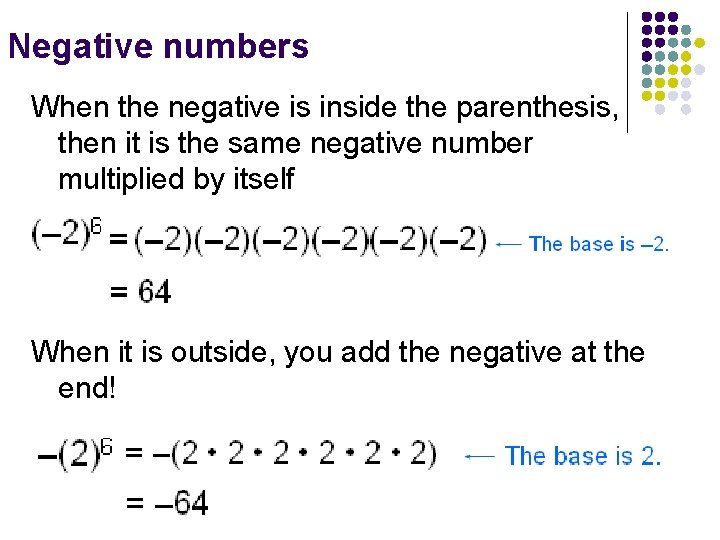Negative numbers When the negative is inside the parenthesis, then it is the same negative number multiplied by itself When it is outside, you add the negative at the end!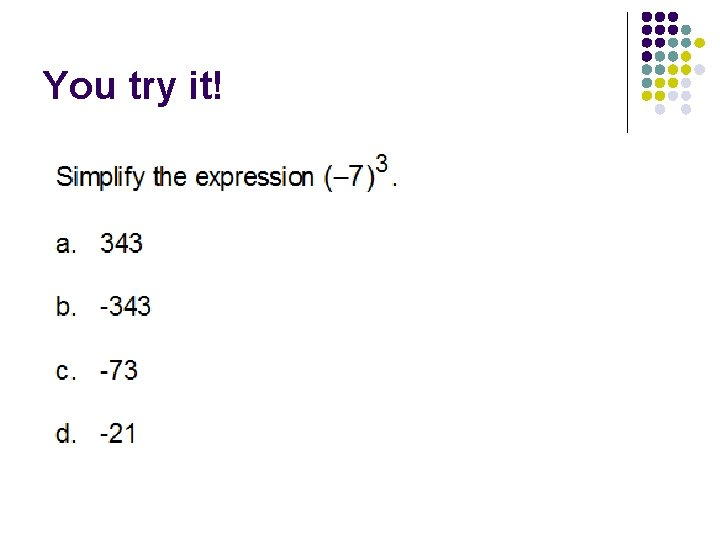You try it!This time it’s different!Example: Write using exponents: a∙b∙b∙b∙a a. b. c. d. 3 2 a b 2 3 a b 2 2 a b aabbbAnd again! x ∙ y ∙ x ∙ x∙ x ∙ y ∙ x a. b. c. d. 6 2 xy x 5 y 2 6 x 2 y 2 5 xy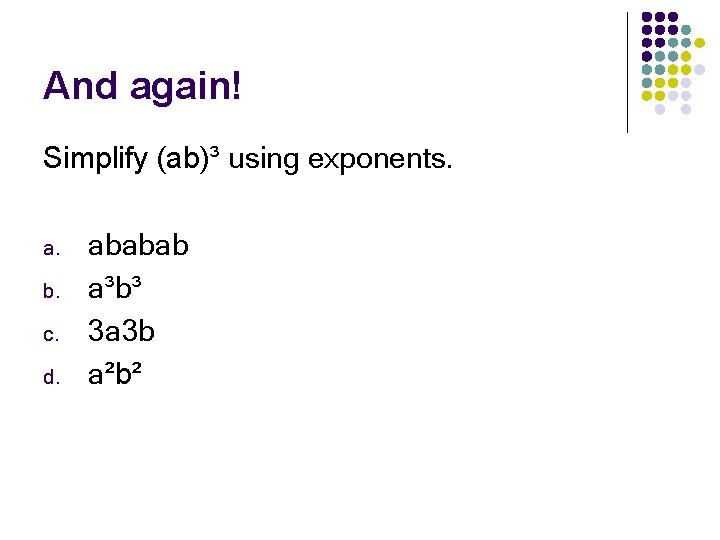And again! Simplify (ab)³ using exponents. a. b. c. d. ababab a³b³ 3 a 3 b a²b²Negative Exponents -n a = Example: 1 n a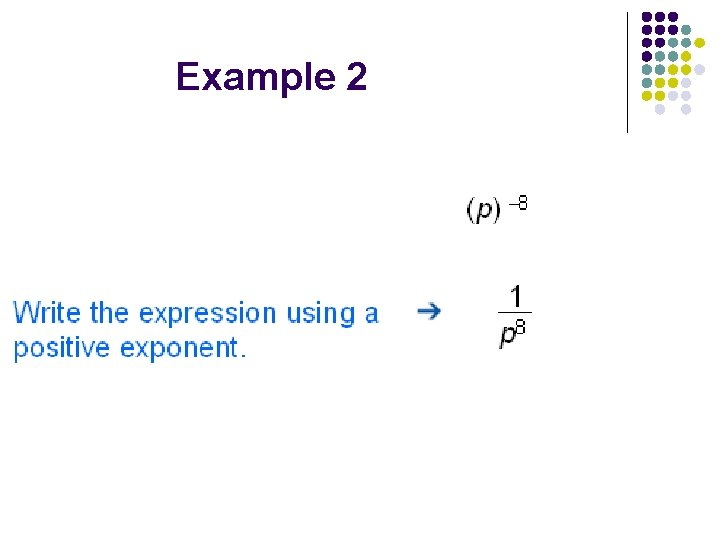Example 2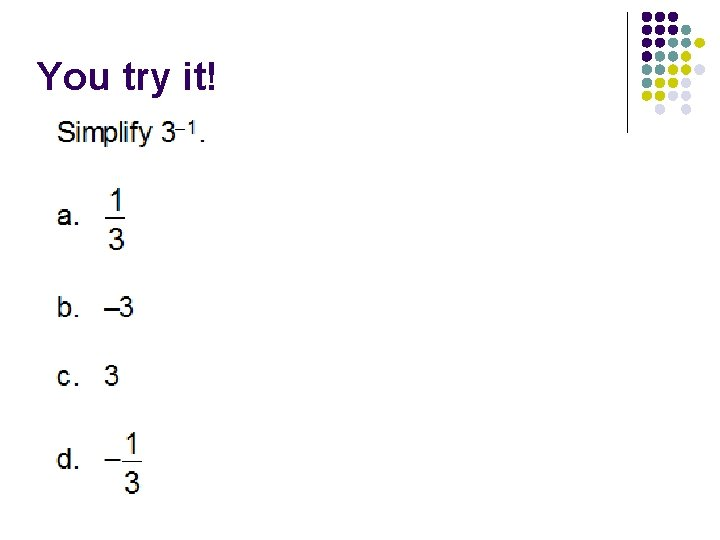You try it!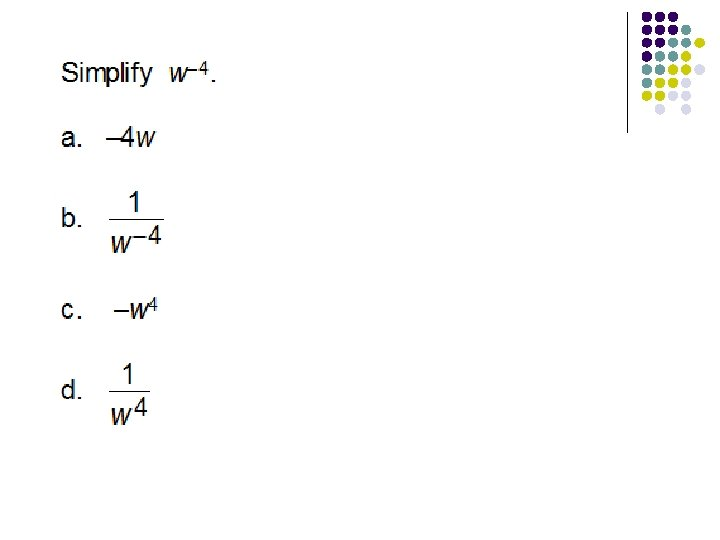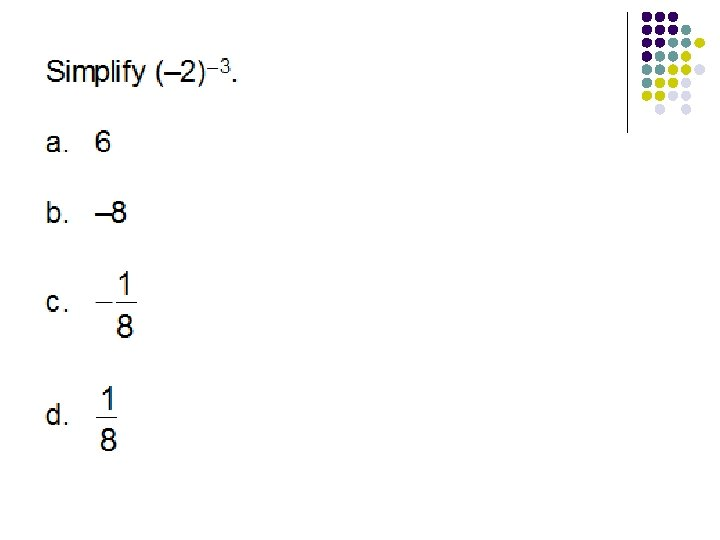Problem of the Day (Thursday) 4 What is (-12) ? a. b. c. d. 20736 -20736 48 -48Exponents – Review!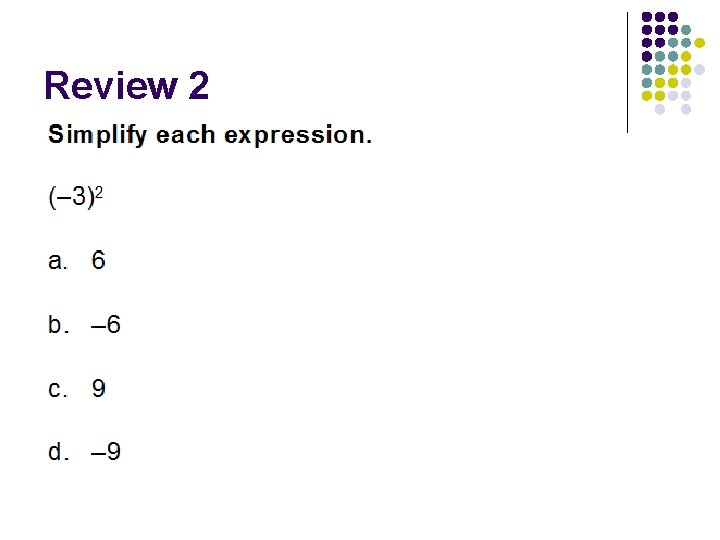Review 2Review 3Review 4 -2 What is 5 ? a. -25 b. 25 c. 1 25 d. 1 -25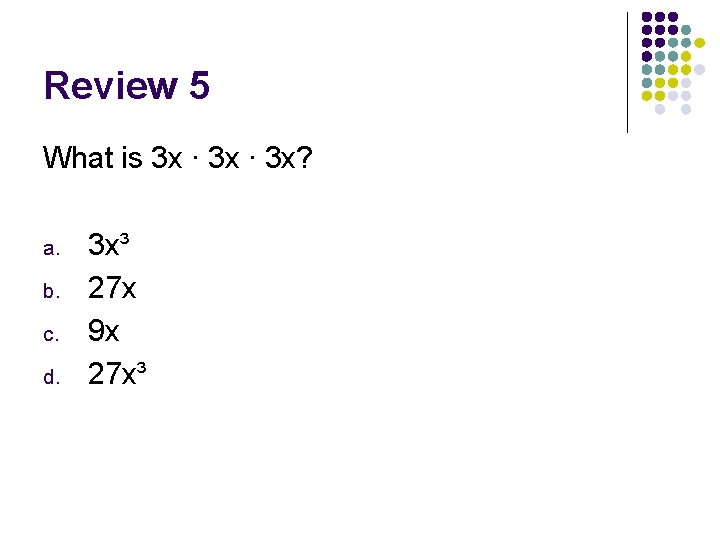Review 5 What is 3 x ∙ 3 x? a. b. c. d. 3 x³ 27 x 9 x 27 x³Multiplying Exponents To multiply numbers or variables with the same base, add the exponents Example: 3³ ∙ 3² = 3∙ 3∙ 3= (3+2) 3 = 35 = 243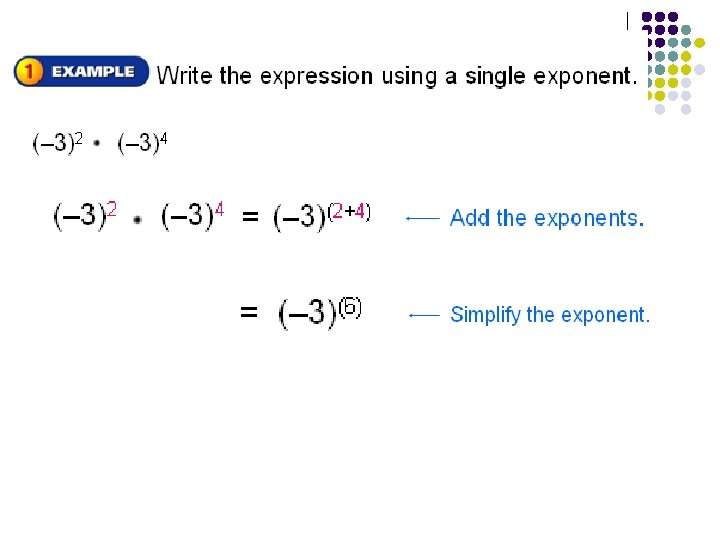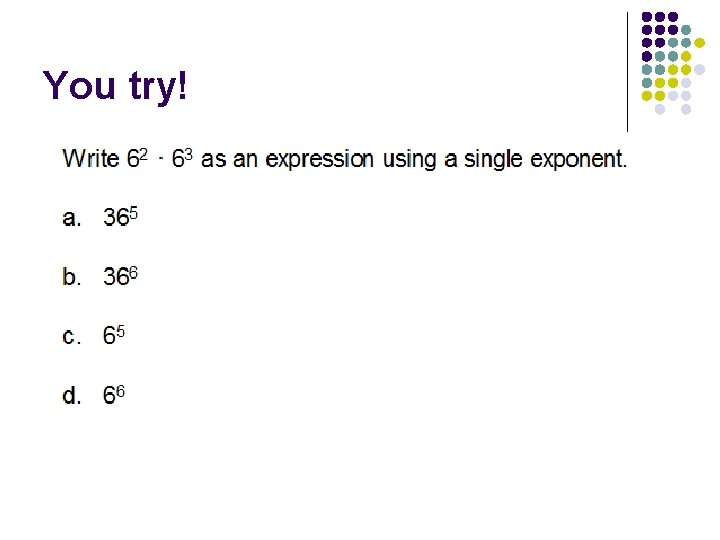You try!Try again!And again!Dividing Exponents When dividing exponents, you subtract! 7 3 3² 3 (7 -2) 5 =3Example 2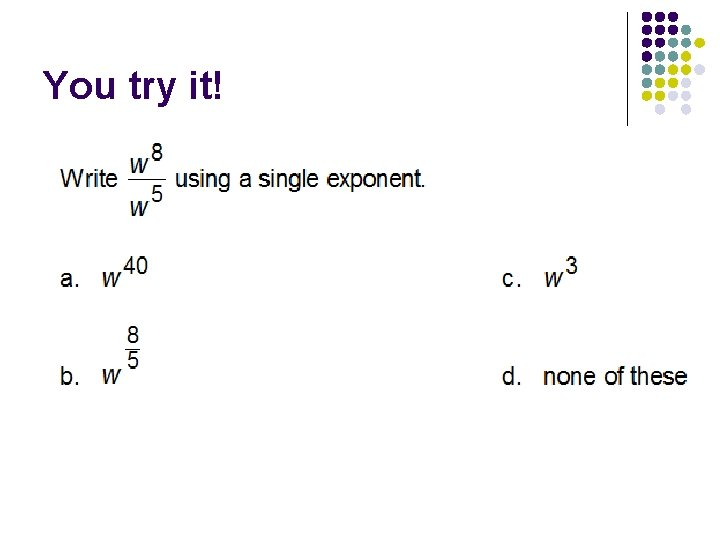You try it!And again! 99 Solve (-7) 98 (-7) a. -7 b. 1 c. 7 d. 7 97And again! 4 Solve w w 6 a. w² b. -2 w c. w 2 d. 1 w²Exponents Raised to a Power Example: (2⁵)² *Multiply the exponents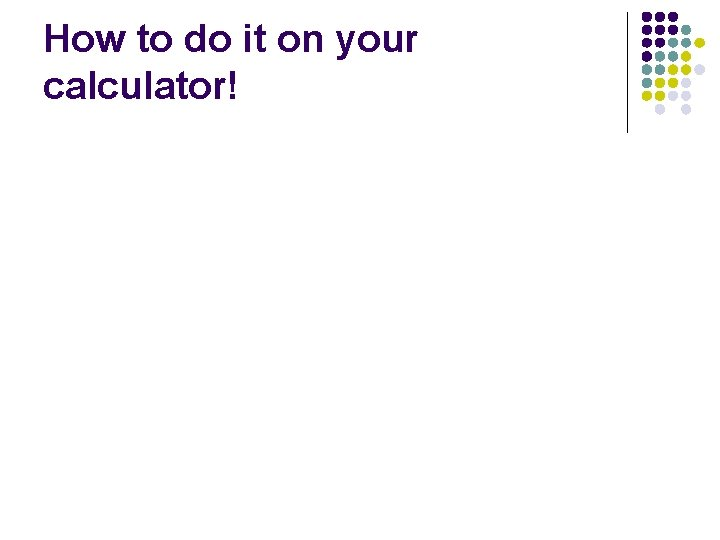How to do it on your calculator!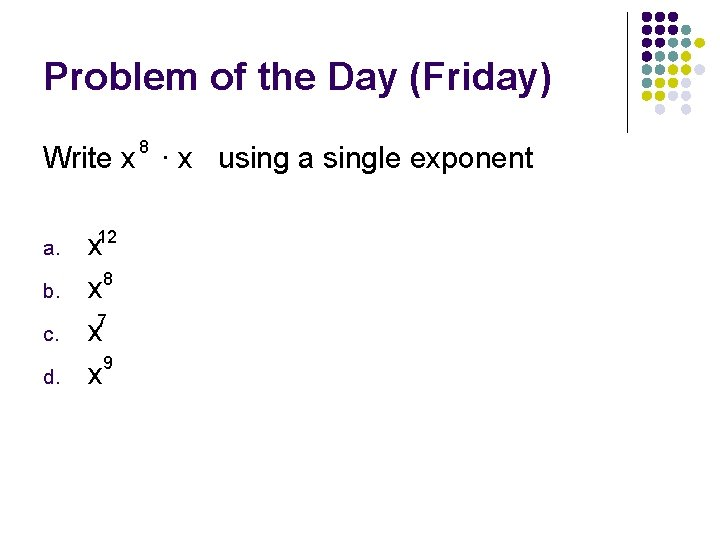Problem of the Day (Friday) 8 Write x ∙ x using a single exponent a. b. c. d. x 12 x 8 7 x 9 x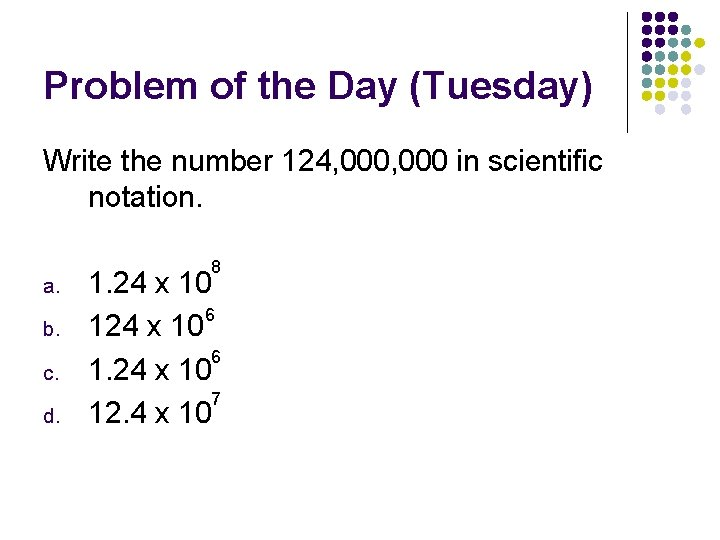Problem of the Day (Tuesday) Write the number 124, 000 in scientific notation. a. b. c. d. 8 1. 24 x 10 6 1. 24 x 10 7 12. 4 x 10Problem of the Day (Tuesday) Write the following using a positive exponent: 6 2 ∙ 6 -5 a. 6³ b. 1_ 6³ c. 1_ 5 6 d. 6 5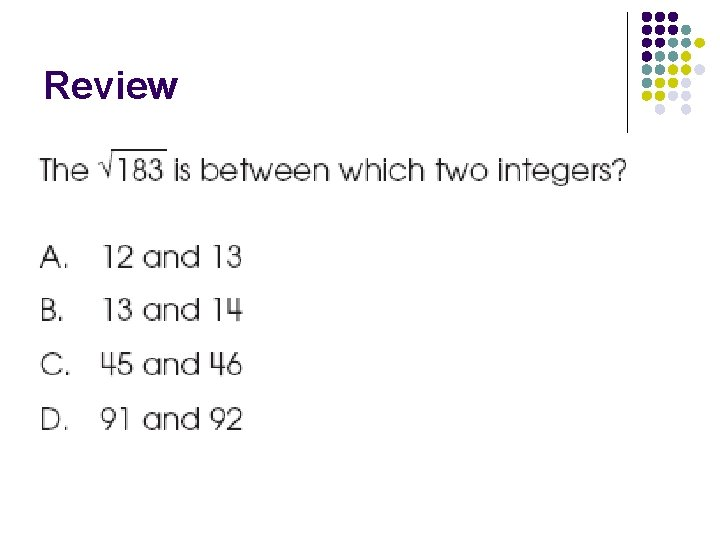ReviewReview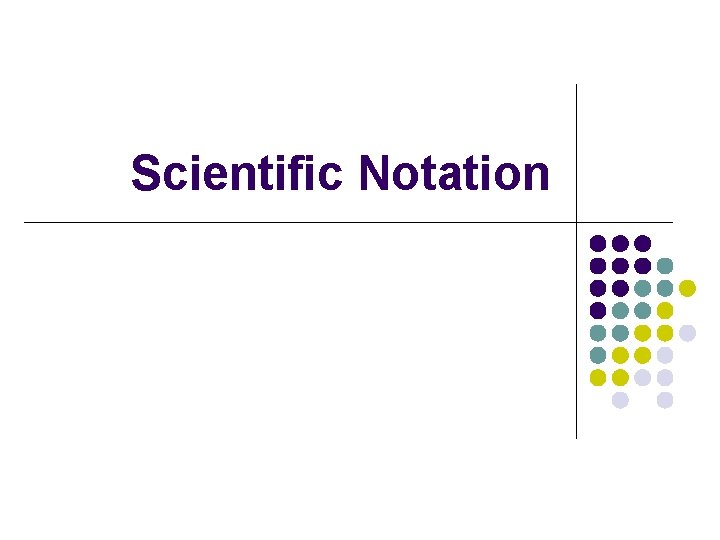Scientific Notation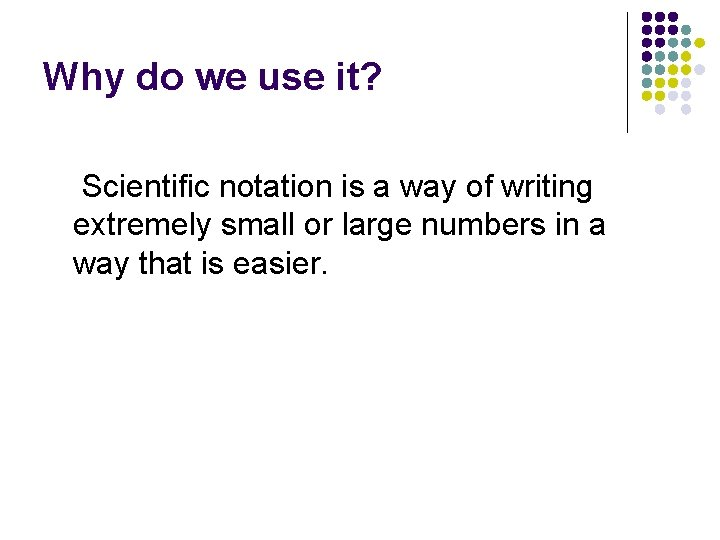Why do we use it? Scientific notation is a way of writing extremely small or large numbers in a way that is easier.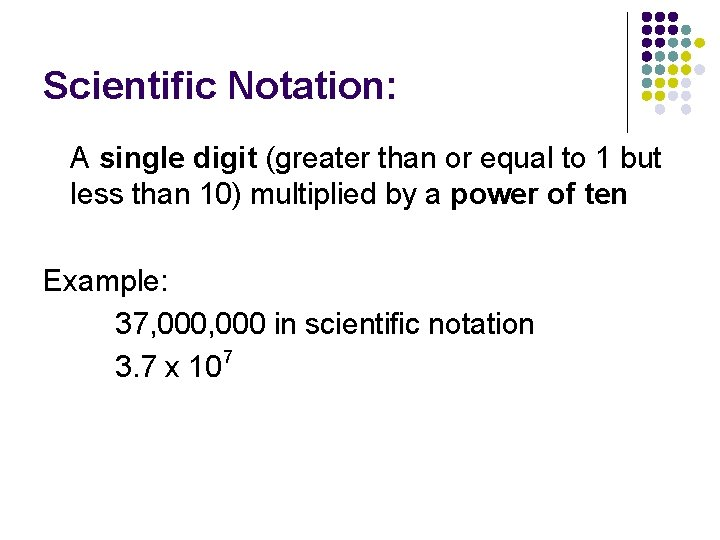Scientific Notation: A single digit (greater than or equal to 1 but less than 10) multiplied by a power of ten Example: 37, 000 in scientific notation 7 3. 7 x 10The other way around 6 1. 55 x 10 in standard form 1, 550, 000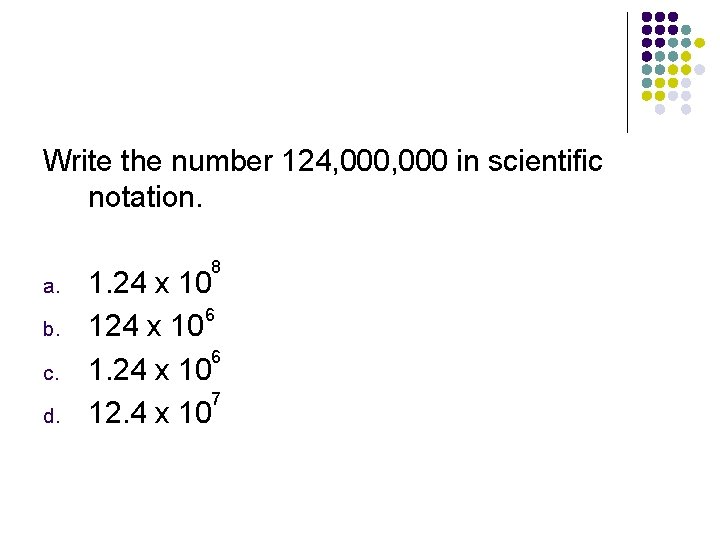Write the number 124, 000 in scientific notation. a. b. c. d. 8 1. 24 x 10 6 1. 24 x 10 7 12. 4 x 10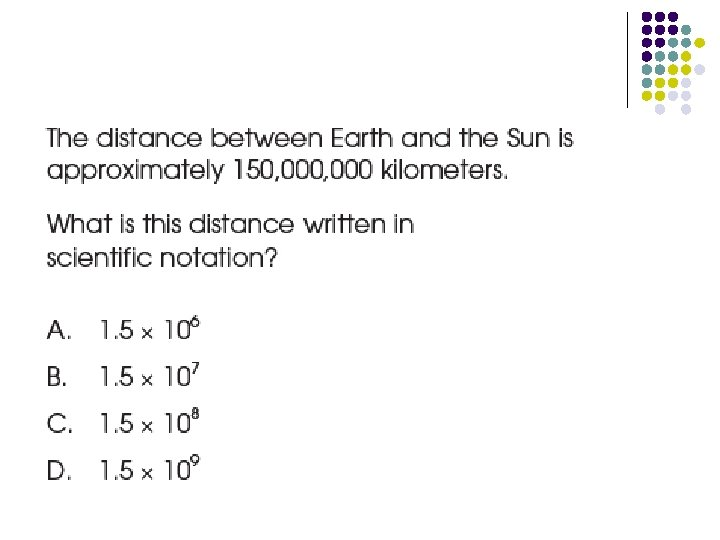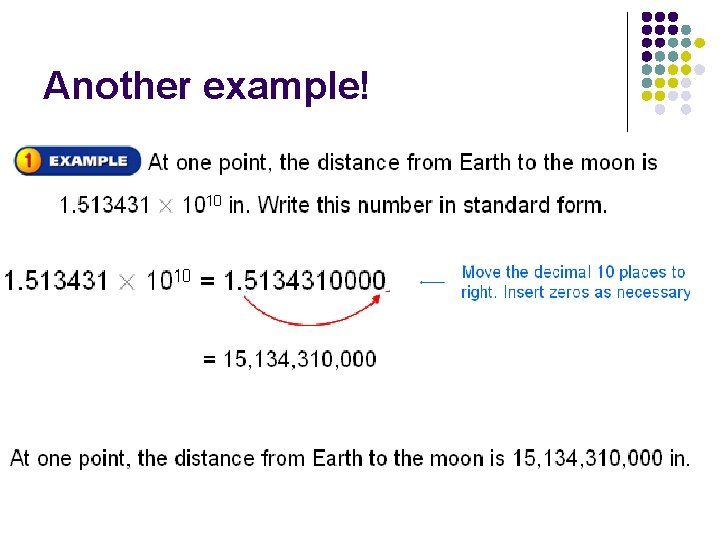Another example!You try it!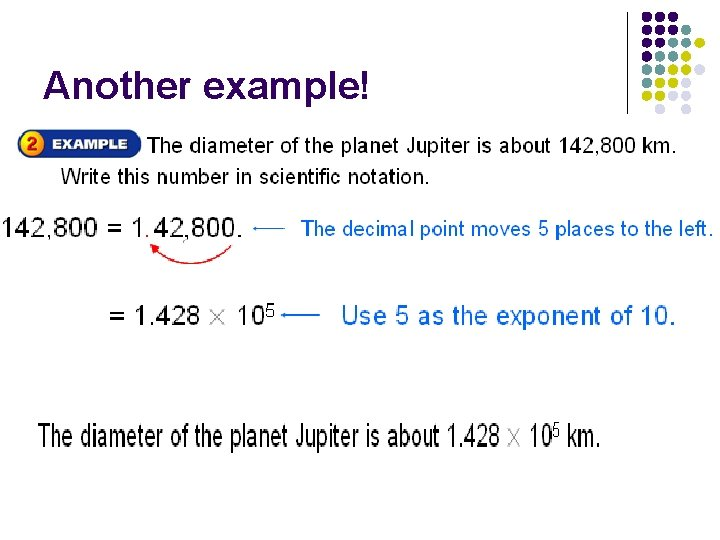Another example!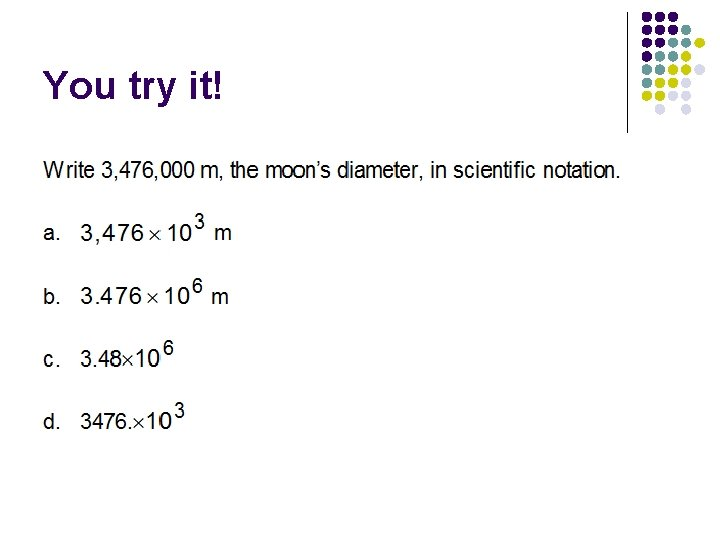You try it!If it is a number less than one: Use negative exponents! Example: 0. 000098 In scientific notation 9. 8 x 10 -5Example 2You try it!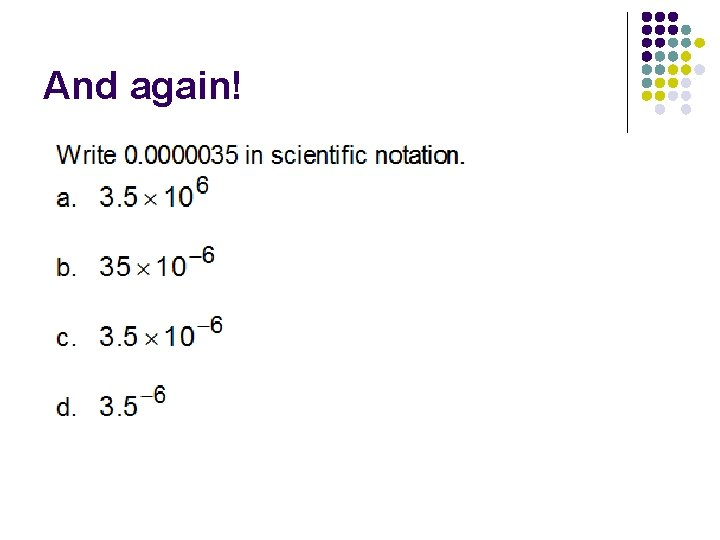And again!Try with a partnerTry with a partner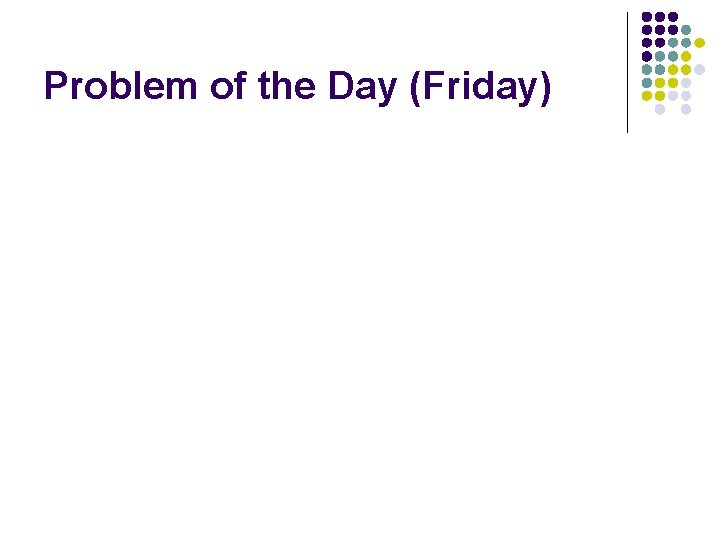Problem of the Day (Friday)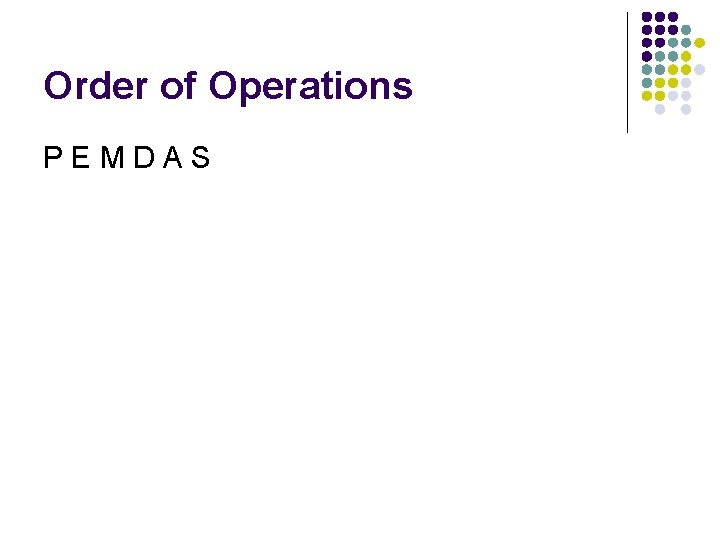Order of Operations PEMDAS